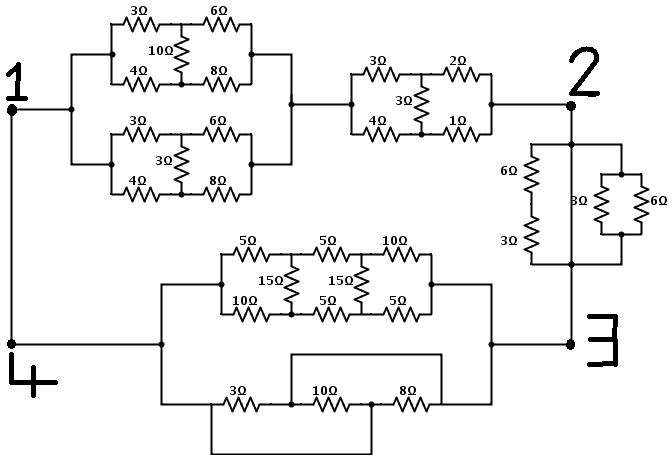# Equivalent Resistance (H)

What is the equivalent resistance (in Ω) between $1$ and $3$ in this circuit, to 2 decimal places?×